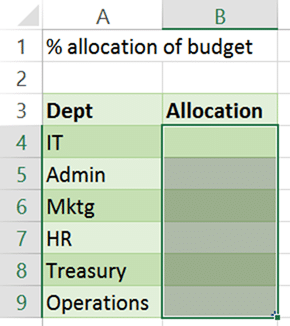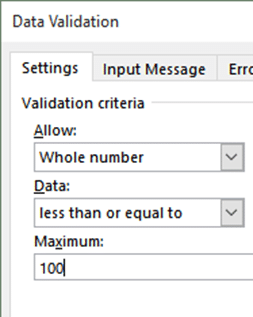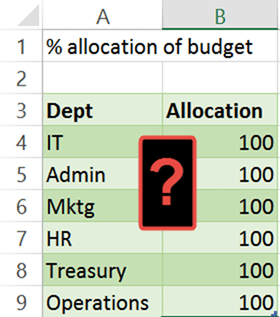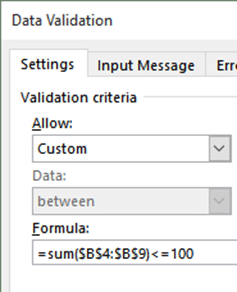## Data Validation using Formula

This is the continuation of the earlier article about Validations. Data Validation using Formula is not very well understood. Hence this article. Try it out and you will immediately know where you can use it. Reading time 9 min.## The Need

Validation is added in Excel in order to prevent data entry mistakes. We select cells and add some validation. The issue is that, each validation rule is applied to individual cell.

What if I want to check for validation across multiple cells? For example, I want to allocate my budget across various departments. The total of percentage allocation should not exceed 100%.This type of validation is not possible using a simple rule like Value must be less than 100. Let us find out why and also discover the solution to this problem.

## The solution: Data Validation using Formula

If we use this type of validation, it will just not work!What is it not working?
Because the restriction was applied to EACH CELL SEPARATELY.

What we really want is to say that the SUM of all these cells should not be more than 100. That is done with Data Validation using Formula.Now it works exactly as expected. Please note that the range is fixed (uses \$ sign). This is important. Without the dollar sign, the range itself would move downwards and the validation will stop working as expected.## The concept behind validation

This was a specific example. But let us understand the core concept. Validation basically uses a formula internally. Even if we choose the Whole Number, Less than or equal to option, it internally converts it to a formula like:

=b4<=100

If the formula returns true, the data entry is allowed. If the formula returns false, the entry is restricted. In short, it just needs a formula which returns a logical value.

Once you understand this concept, you can write more complex (and more useful) formula based validations.

Try it out and let me know if this was useful.

##### Subscribe to Blog

Join 2,178 other subscribers